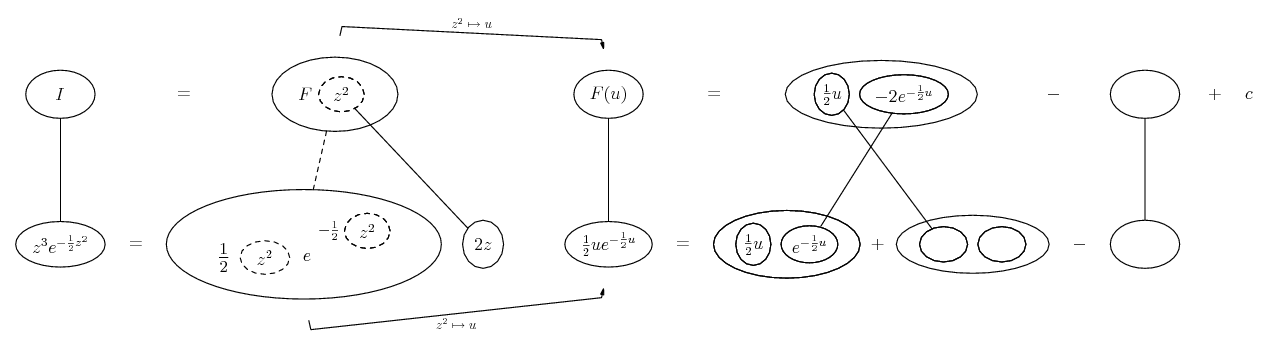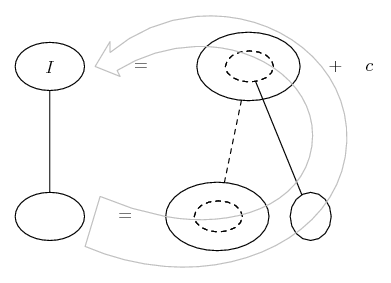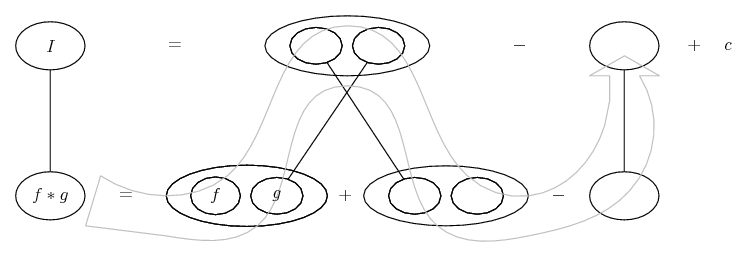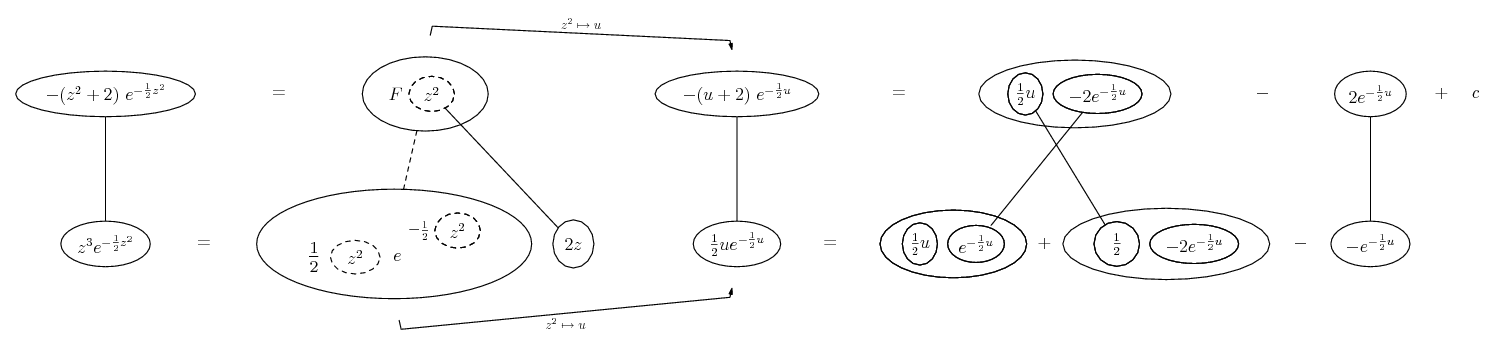# Thread: Proving skewness of normal equals zero by doing the integration

1. ## Proving skewness of normal equals zero by doing the integration

Hey guys,

I know the skewness of a standard normal distribution must be zero, but in order to prove this I need to integrate (from negative to positive infinity):

z^3*e^((-z^2)/2)

I know a result for integrating e^(-az^2), but not sure how to handle things when it's multiplied by z^3.

Any advice would be much appreciated.

Cheers!

2. u = z^2, then integrate $\displaystyle \frac{1}{2} u\ e^{-\frac{1}{2} u}$ by parts

Edit:

Just in case a picture helps (spoiler because of width)...

Spoiler:... where... is the chain rule. Straight continuous lines differentiate downwards (integrate up) with respect to z, and the straight dashed line similarly but with respect to the dashed balloon expression (the inner function of the composite which is subject to the chain rule).

The general drift is...Except we also detour through parts...This spoiler in case of fun...
Spoiler:_________________________________________

Don't integrate - balloontegrate!

Balloon Calculus; standard integrals, derivatives and methods

Balloon Calculus Drawing with LaTeX and Asymptote!

3. Wow, thanks for the in-depth response.

#### Search Tags

equals, integration, normal, proving, skewness